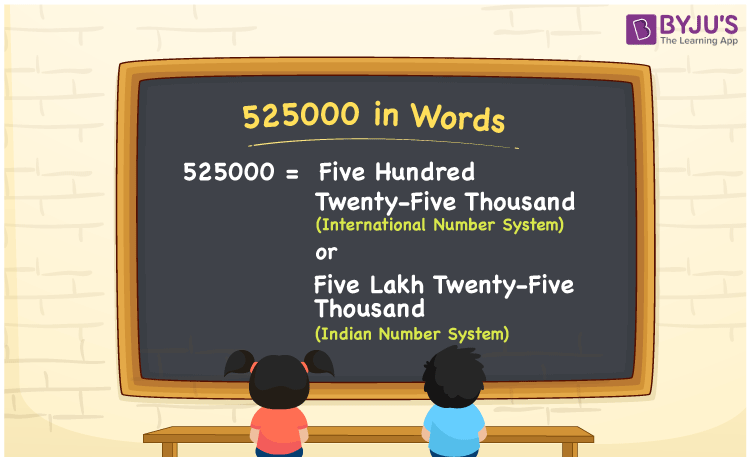# 525000 in Words

We can write 525000 in words as Five Hundred Twenty-Five Thousand (International Number System) or Five Lakh Twenty-Five Thousand (Indian Number System).For example, Arun applied for a personal loan of Rs. 525000, then you can express, “Arun applied for a personal loan of Rupees Five Lakh Twenty-Five Thousand”. Place value table is very useful in expressing the numerical name of any number easily. We know that 525000 is a cardinal number as it indicates an exact value. With the help of a place value chart, let us learn the method of converting the number 525000 into words in this article.

 525000 in words International Number System Indian Number System Five Hundred Twenty-Five Thousand Five Lakh Twenty-Five Thousand Five Hundred Twenty-Five Thousand or Five Lakh Twenty-Five Thousand in numerical form 525000

## 525000 in English Words

English is a vital language that is used in education worldwide. Hence, we write numbers in words with the help of the English alphabet. Therefore, we can spell 525000 in English words as Five Hundred Twenty-Five Thousand or Five Lakh Twenty-Five Thousand.## How to Write 525000 in Words?

Place value chart plays a vital role in expressing the numbers into words. Here, the number 525000 is a six-digit number. Hence, we should prepare a table of 6 columns to indicate its place value chart. This can be shown as follows.

 Lakhs Ten-Thousands Thousands Hundreds Tens Ones 5 2 5 0 0 0

Hence, we can write the expanded form as:

5 x Lakh + 2 x Ten Thousand + 5 x Thousand + 0 x Hundred + 0 x Ten + 0 x One

= 5 x 100000 + 2 x 10000 + 5 x 1000 + 0 x 100 + 0 x 10 + 0 x 1

= 500000 + 20000 + 5000 + 0 + 0 + 0

= 500000 + 20000 + 5000

= 525000

= Five Hundred Twenty-Five Thousand or Five Lakh Twenty-Five Thousand

Therefore, 525000 in words is written as Five Hundred Twenty-Five Thousand or Five Lakh Twenty-Five Thousand

Interesting way of writing 525000 in words

5 = Five

52 = Fifty-Two

525 = Five Hundred and Twenty-Five

5250 = Five Thousand Two Hundred Fifty

52500 = Fifty-Two Thousand Five Hundred

525000 = Five Hundred Twenty-Five Thousand or Five Lakh Twenty-Five Thousand

Thus, the word form of the number 525000 is Five Hundred Twenty-Five Thousand or Five Lakh Twenty-Five Thousand

525000 is a natural number that precedes 525001 and succeeds 524999

• 525000 in words – Five Hundred Twenty-Five Thousand or Five Lakh Twenty-Five Thousand
• Is 525000 an odd number? – No
• Is 525000 an even number? – Yes
• Is 525000 a perfect square number? – No
• Is 525000 a perfect cube number? – No
• Is 525000 a prime number? – No
• Is 525000 a composite number? – Yes

## Frequently Asked Questions on 525000 in Words

Q1

### How do you write 525000 in words?

We can write 525000 in words as Five Hundred Twenty-Five Thousand or Five Lakh Twenty-Five Thousand.
Q2

### What is the value of 400000 + 125000 in words?

400000 + 125000 = 525000. Hence, the value of 400000 + 125000 in word form is Five Hundred Twenty-Five Thousand or Five Lakh Twenty-Five Thousand.
Q3

### Is 525000 a prime number?

No, the number 525000 is not a prime number.